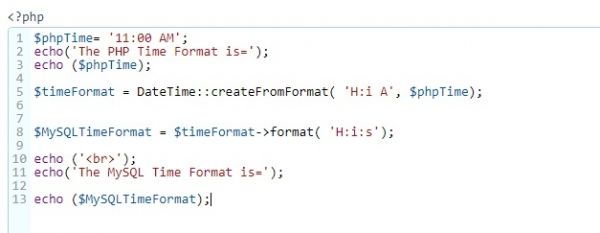# Convert PHP variable “11:00 AM” to MySQL time format?

MySQLMySQLi Database

Use DateTime to convert PHP variable “11:00 AM: to MySQL time format.

The PHP code is as follows −

$phpTime = '11:00 AM'; echo('The PHP Time Format is ='); echo ($phpTime);
$timeFormat = DateTime::createFromFormat( 'H:i A',$phpTime);
$MySQLTimeFormat =$timeFormat->format( 'H:i:s');
echo ('
');
echo('The MySQL Time Format is =');
echo (\$MySQLTimeFormat);

The snapshot of PHP code is as follows −Here is the output −

The PHP Time Format is =11:00 AM
The MySQL Time Format is =11:00:00